• #include <stdio.h> #define n 10 ... //标记数组 for(int i = 0; i < n; i++){ //输入 scanf("%d",&a[i]); } int *p[n]; for(int i = 0; i < n; i++) { //使指...

#include <stdio.h>
#define n 10
int max(int a[],int b[],int );

int main() {
int a[n] = {0};
int b[n] = {0};							//标记数组

for(int i = 0; i < n; i++){				//输入
scanf("%d",&a[i]);
}

int *p[n];
for(int i = 0; i < n; i++) {			//使指针指向对应的元素
p[i] = &a[i];
}

for(int i = 0; i < n; i++) {			//每次指向当前剩余元素中的最大值；
int max_i = max(a,b,n);

p[i] = &a[max_i];
b[max_i] = 1;						//最大值标记为 1
}

for(int i = 0; i < n; i++){				//输出
printf("%d ",*p[i]);
}

return 0;
}

int max( int a[], int b[], int len ){       //返回b数组中未被标记的最大值的下标
int max = -999999999,k = 0;				//保证在 > -999999999 的范围内，所有数都可排序
for(int i = 0; i < len; i++){
if(b[i] == 0 && a[i] > max){		//有标记的数字不再作为最大值
max = a[i];
k = i;
}
}
return k;
}

展开全文排序算法
• 一、数组说动态数组之前，首先要说数组数组是一种顺序存储的线性表，所有元素的内存地址都是连续的。数组的最大优点是他的查找时间复杂度能够达到O(1)，但是增和删的时间复杂度较高O(n)二、动态数组动态数组，即...

一、数组

说动态数组之前，首先要说数组，数组是一种顺序存储的线性表，所有元素的内存地址都是连续的。数组的最大优点是他的查找时间复杂度能够达到O(1)，但是增和删的时间复杂度较高O(n)

二、动态数组

动态数组，即根据用户的输入动态扩充或缩小当前数组的容量。在python中，已经内置了动态数组，叫做列表，List

下面是利用python代码实现动态数组的增删改查操作。

# ArrryList.py

class Arr:

# 设置两个常亮，分别是默认容量大小与未找到元素返回的值

DEFAULT_CAPACITY = 10

ELEMENT_NOT_FOUND = -1

# 初始化，若用户未指定数组大小或者小于默认，直接使用默认容量，否则容量为用户指定

def __init__(self, capacity=DEFAULT_CAPACITY):

capacity = self.DEFAULT_CAPACITY if capacity < self.DEFAULT_CAPACITY else capacity

self._capacity = capacity

self._size = 0

self._elements = [None] * self._capacity

def size(self):

return self._size

def is_empty(self):

# return True if self._size == 0 else False

# 改进上面这还代码，因为在python中所有值都可以转布尔，所以利用隐式布尔值

return self._size == 0

# 查看元素是否存在--->bool

def contains(self, element):

# if self.index_of(element):

# return True

# return False

return True if self.index_of(element) else False

# 根据索引添加，添加结束后判断是否需要扩容

self._out_of_index(index)

self._size = i = self._size + 1

while i >= index:

self._elements[i] = self._elements[i - 1]

i -= 1

self._elements[index] = element

self._expend_capacity()

# 数组末尾追加，添加结束后判断是否需要扩容

self._out_of_index(self._size)

self._elements[self._size] = element

self._size += 1

self._expend_capacity()

# 根据索引取值

def get(self, index):

self._out_of_index(index)

return self._elements[index]

# 根据索引改值

def set(self, index, element):

self._out_of_index(index)

self._elements[index] = element

# 删除元素，若用户未指定参数，默认删除最后一个元素，删除后判断是否要缩容

def remove(self, index=ELEMENT_NOT_FOUND):

self._out_of_index(index)

if index == self.ELEMENT_NOT_FOUND:

self._remove_last()

# 删除元素后，该索引后的每个元素都要往前移一格，然后数组大小减一

i = index

while i <= self._size:

self._elements[i] = self._elements[i + 1]

i += 1

self._size -= 1

self._reduce_capacity()

# 返回第一个匹配传入值的索引

def index_of(self, element):

for index in range(self._size + 1):

if element == self._elements[index]:

return index

return self.ELEMENT_NOT_FOUND

def clear(self):

self._size = 0

return self._size

# 判断索引是否越界

def _out_of_index(self, index):

if index < 0 or index > self._size + 1:

raise IndexError('index out of range')

# 当容量不够时动态扩容，默认为扩大为原来的1.5倍

def _expend_capacity(self):

# 当size小于容量，直接返回，当size正好等于容量，需要扩容

if self._size < self._capacity - 1:

return

self._capacity = self._capacity * 2

self._new_elements = [None] * self._capacity

for i in range(self._size):

self._new_elements[i] = self._elements[i]

self._elements = self._new_elements

# 动态缩容，默认缩小到当前的一半

def _reduce_capacity(self):

# 当size小于默认容量或者大于当前容量的一半时直接返回

if self._size <= self._capacity or self._size > (self._capacity // 2):

return

self._capacity = (self._capacity // 2).__ceil__()

for i in range(self._size):

self._new_elements[i] = self._elements[i]

self._elements = self._new_elements

def __str__(self):

arrlist = ''

num = 0

for i in self._elements:

if num == self._size:

break

arrlist = arrlist + str(i) + ','

num += 1

arrlist = arrlist.strip(",")

arrlist = '[' + arrlist + ']'

return arrlist

"""

删除最后一个元素，因为最后一个元素也是往前移动一格，虽然size-1，但是其实最后一个元素引用了两次，

即当前的数组末尾加上原来位置的引用，无法回收，所以对于最后一个元素要手动设置为None

"""

def _remove_last(self):

self._size -= 1

self._elements[self._size] = None

self._reduce_capacity()

# 测试文件,test.py

if __name__ == '__main__':

from ArrayList import Arr

a = Arr()

# a = a #type:Arr

# a.remove(2)

# print(a.size())

# print(a.is_empty())

# print(a.contains('55'))

# print(a.index_of(99))

# print(a.get(789))

# a.set(-1,99)

# a.clear()

print(a)

展开全文• printf("请输入字符：\n"); for(i=0;i;i++) scanf("%c",&a[i]); for(i=0;i;i++) Push(&S,a[i]); for(i=0;i;i++) Pop(&S,&a[i]); for(i=0;i;i++) printf("%c",a[i]); } int InitStack(SqStack *S) {S->base=(char *)...

该楼层疑似违规已被系统折叠 隐藏此楼查看此楼

#include"stdio.h"

#include"stdlib.h"

#define STACK_INIT_SIZE 100

#define STACKINCREMENT 10

typedef struct

{char *base;

char *top;

int stacksize;

}SqStack;

main()

{SqStack S;

char a;

int i;

InitStack(&S);

printf("请输入字符：\n");

for(i=0;i<4;i++)

scanf("%c",&a[i]);

for(i=0;i<4;i++)

Push(&S,a[i]);

for(i=0;i<4;i++)

Pop(&S,&a[i]);

for(i=0;i<4;i++)

printf("%c",a[i]);

}

int InitStack(SqStack *S)

{S->base=(char *)malloc(STACK_INIT_SIZE * sizeof(char));

if(!S->base) return 0;

S->top=S->base;

S->stacksize=STACK_INIT_SIZE;

return 1;

}

int Push(SqStack *S,char e)

{if(S->top-S->base>=S->stacksize)

{S->base=(char *)realloc(S->base,(S->stacksize+STACKINCREMENT) * sizeof(char));

if(!S->base) return 0;

S->top=S->base+S->stacksize;

S->stacksize+=STACKINCREMENT;

}

*S->top++=e;

return 1;

}

int Pop(SqStack *S,char *e)

{if(S->top==S->base) return 0;

*e=*--S->top;

return 1;

}

展开全文• 第六章数组 数组：一批具有同名的同属性的数据 （1）数组是一组有序数据的集合 （2）用一个数组名及下标确定元素 （3）数组中每个元素属于同一数据类型 定义数组 类型符 数组名【常量表达式】 怎样引用一组数据元素 ...

第六章数组

数组：一批具有同名的同属性的数据
（1）数组是一组有序数据的集合
（2）用一个数组名及下标确定元素
（3）数组中每个元素属于同一数据类型

定义数组
类型符 数组名【常量表达式】

怎样引用一组数据元素
数组名[下标]

用数组处理斐波那契数列问题

#include<stdio.h>
int main()
{
int i;
int f = {1,1};
for(i=2;i<20;i++)
{
f[i] = f[i-1] + f[i-2];
}
for(i=0;i<20;i++)
{
printf("%d\n",f[i]);
}
return 0;
}

冒泡排序

#include<stdio.h>
int main()
{
int a; //= {34,67,90,43,124,87,65,99,132,26};
int i,j,t;

for(i=0;i<10;i++)
{
scanf("%d",&a[i]);
}

for(j=0;j<9;j++){
for(i=0;i<9-j;i++)
{
if(a[i]>a[i+1])
{
t = a[i];
a[i] = a[i+1];
a[i+1] = t;
}
}
}

for(i=0;i<10;i++)
{
printf("%d\n",a[i]);
}
return 0;
}

二维数组常称为矩阵，把二维数组写成行和列的排列形式
怎样定义二维数组
类型说明符 数组名【常量表达式】【常量表达式】

怎样引用二维数组的元素
二维数组元素的表现形式为：数组名【下标】【下标】

#include<stdio.h>
int main()
{
int a = {{1,2,3},{4,5,6}};
int b;
int i,j;
for(i=0;i<=1;i++)
{
for(j=0;j<=2;j++)
{
printf("%5d",a[i][j]);
b[j][i] = a[i][j];
}
printf("\n");
}
printf("\n");

for(i=0;i<3;i++)
{
for(j=0;j<2;j++)
{
printf("%5d",b[i][j]);
//printf("\n");
}
printf("\n");
}
return 0;
}

#include<stdio.h>
int main()
{
int a = {{1,2,413,3},{4,77,4,3},{9,7,5,6}};
int i,j;
int t = 0;
int h=0,l=0;
for(i = 0;i<3;i++)
for(j=0;j<4;j++)
{
if(a[i][j]>t)
{
t = a[i][j];
h = i;
l = j;
}
}
printf("%d\n%d\n%d\n",t,h,l);

return 0;
}

C存放字符串变量的方式
1.字符数组
2.字符型指针

怎样定义字符数组

用来存放字符数据的是字符数组
在字符数组内的一个元素存放一个字符
定义字符数组的方法与定义数值型数组的方法类似
字符型数据是以整数形式（ASCII码）存放的

（1）字符串常量最后有一个‘\0’
（2）“”等价于‘\0’，是一个空字符串

字符数组的输入输出

1.逐个字符输入输出 用格式符号%c输入或者输出一个字符
2.将整个字符串一次输入或者输出，用格式符号%s，意思是对字符创string的输入输出 输出时遇到’\0’就结束
输出的字符不包括‘\0’
%s格式输出字符串时，printf函数中输出项是字符数组名，不是数组元素名
若一个数组包含一个以上‘\0’则遇到第一个‘\0’时输出就结束

使用字符串处理函数

puts函数 字符数组
Gets 输入字符串的函数 从终端输入一个字符串到字符数组
Strcat函数 字符串连接函数
Strcat（字符数组1，字符数组2）
1.字符数组1必须足够大
2.连接两个字符数组的后面都有‘\0’,连接时将字符数组1后面的‘\0’取消，只在新串最后保留‘\0’
Strcpy和strncpy函数
Strcpy（字符数组1，字符串2） 字符串复制函数，将字符串2复制到字符数组1中去
Strncpy函数将字符串2前面的n个复制到字符串1中去 strncpy(str1,str2,2)
strcmp函数 字符串比较函数
Strcmp（字符串1，字符串2）
比较规则：将两个字符串自左向右逐个字符相比（按ASCII码值大小比较）直到出现不同的字符或者遇到‘\0’为止
1.如果出现全部字符相同 则两个字符串相等
2.若出现不相同字符，则以第一对不相同的字符的比较结果为准
英文字典中位置在后的大 小写字母比大写字母大
字符串1=字符串2 0
若> 正
若< 负
Strlen函数
strlen（字符数组）//测试字符串长度的函数 函数的值为字符串的实际长度 不包括‘\0’
strlwr转换小写函数
strlwr（字符串）//将字符串中大写字母换成小写字母
Strupr 转换大写函数

统计有多少个单词

#include<stdio.h>
int main()
{
char a;
gets(a);
int i;
int num=0;
for(i=0;  i<=100;i++)
{
if(a[i] != ' ' && a[i-1] == ' ')
{
num++;
}
}
printf("%d\n",num);
return 0;
}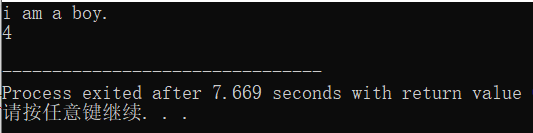有三个字符串，要求找出其中最大者

#include<stdio.h>
#include<string.h>
int main()
{
char str1;
char str2;
char str3;
char str4;
gets(str1);
gets(str2);
gets(str3);
printf("\n");
int c;
c = strcmp(str1,str2);
if(c>0)
strcpy(str4,str1);
else strcpy(str4,str2);
c = strcmp(str4,str3);
if(c>0)
strcpy(str4,str4);
else strcpy(str4,str3);
puts(str4);
return 0;
} //书上的更加简洁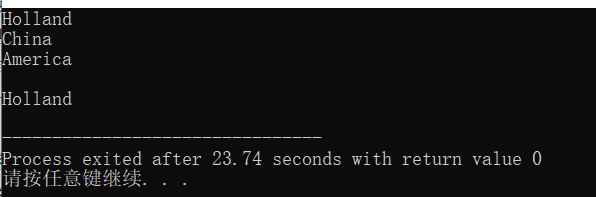第六章课后习题

1.用筛选法求100以内素数

#include<stdio.h>
#include<string.h>
#include<math.h>
int main()
{
char a;
int i,j;
for(i=1;i<=100;i++)
{
a[i] = i;
}
a = 0;
for(i = 2;i<=sqrt(100);i++)
{
for(j = i+1;j<=100;j++)
if(a[i] != 0 && j != 0 && a[j] % i == 0)
{
a[j] = 0;
}
}
for(i = 1;i<=100;i++)
{
if(a[i] != 0)
{
printf(" %d",a[i]);
}
}
return 0;
}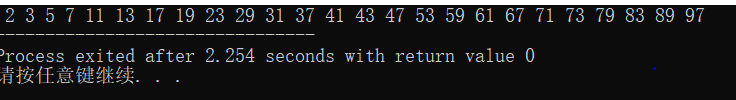2.用选择法对10个整数排序

#include<stdio.h>
#include<string.h>
#include<math.h>
int main()
{
int i, j,a,t,m;
for( i = 1;i<=10;i++)
{
printf("a[%d]=",i);
scanf("%d",&a[i]);
}
printf("\n");
for(i = 1;i<=9;i++)
{
m=i;
for(j = i+1;j<=10;j++)
{
if(a[m]>a[j]) m = j;
}
t = a[i];
a[i] = a[m];
a[m] = t;
}
for(i=1;i<=10;i++)
{
printf(" %d",a[i]);
}
return 0;
}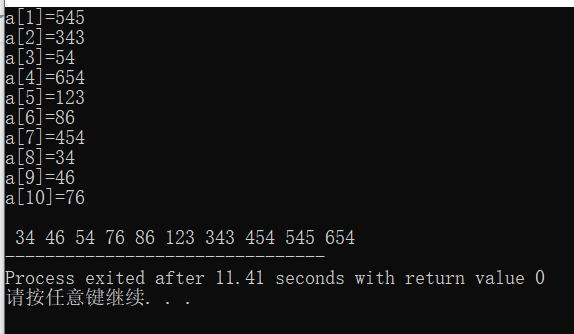3.求一个3X3的整型矩阵对角线元素之和

#include<stdio.h>
#include<string.h>
#include<math.h>
int main()
{
int a;
int i,j,sum=0;
for(i = 0;i<3;i++)
for (j=0;j<3;j++)
{
printf("a[%d][%d]=",i,j);
scanf("%d",&a[i][j]);
}
//sum = a + a +a;
for(i=0;i<3;i++)
{
sum = sum + a[i][i];
}
printf("sum = %d\n",sum);

return 0;
}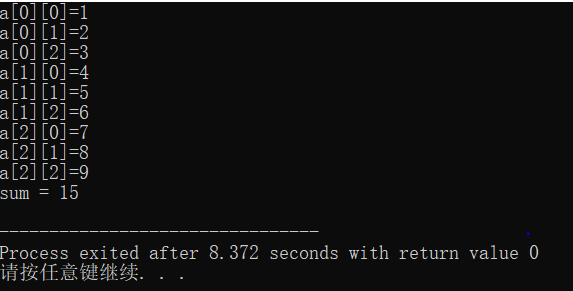4.有一个已经排好序的数组，要求输入一个数字后，按照原来排序的规律将它插入到数组中

#include<stdio.h>
#include<string.h>
#include<math.h>
int main()
{
int a = {1,2,3,4,6,7,8,19,34,56};
int k,i,j,m,n,end;
printf("输入插入的数字：");
scanf("%d",&k);
end = a;
if(k>end) k = a;
else
{
for(i = 0;i<=10;i++)
{
if(k>a[i-1] && k<a[i])
{
m = a[i];
a[i] = k;
//将k的值赋给了a[i]
for(j=i+1;j<=10;j++)
{
n = a[j];
a[j] = m;
m = a[j];
//将i+1及以后的元素右移一个单位
}

break;
}
}
}
for(i=0;i<11;i++)
{
printf(" %d",a[i]);
}
return 0;
}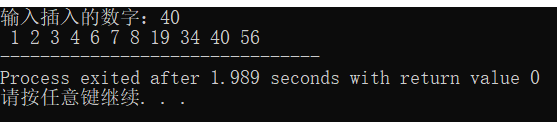5.将一个数组按逆序重新存放，例如，原来顺序 8，6,5,4,1 要求改为1,4,5,6,8

#include<stdio.h>
#include<string.h>
#include<math.h>
int main()
{
int a = {8,6,5,4,1};
int i,j;
for(i=4;i>=0;i--)
{
printf("%d ",a[i]);
}

return 0;
}

这种不对 没有改变存储方式

#include<stdio.h>
#include<string.h>
#include<math.h>
int main()
{
int a;
int i,t;
for(i=1;i<=5;i++)
{
printf("a[%d]=",i);
scanf("%d",&a[i]);
}
printf("\n");
/*for(i=5;i>=1;i--)
{
printf("a[%d]=%d\n",i,a[i]);

}*/
for(i=1  ; i<=5/2;i++)
{
t = a[i];
a[i] = a[5-i+1];
a[5-i+1]=t;

}
for(i=1;i<=5;i++)
{
printf("%d ",a[i]);
}
return 0;
}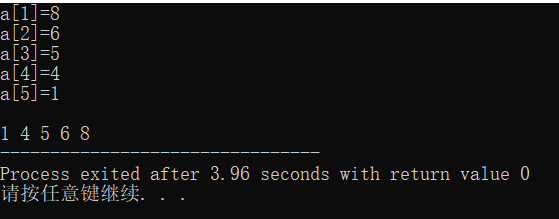展开全文字符串 数据结构 c# 数组 java
• 1. 顺序表的位序和元素下标是两个不同概念，位序从1开始，元素下标从0开始。位置i的元素，它的下标i-1 2.i的值在[1，L->length+1]区间内时，都是有效的插入位置。1表示待插入元素取代第1个元素，L->length...c语言 数据结构 顺序表 线性表
• 所谓数组，就是相同数据类型的元素按一定顺序排列的集合；数组的存储区间是连续的，占用内存比较大，故空间复杂的很大。但数组的二分查找时间复杂度小，都是O(1)；数组的特点是：查询简单，增加和删除困难。 在内存...链表 数据结构
• 数组

2021-03-10 00:56:13
若将有限个类型相同的变量的集合命名，那么这个名称为数组名。组成数组的各个变量称为数组的分量，也称为数组的元素，有时也称为下标变量。用于区分数组的各个元素的数字编号称为下标。数组是在程序设计中，为了...java中数组的含义
• 第六章 利用数组处理批量数据6.1 怎样定义和引用一维数组6.1.1 怎样定义一维数组6.1.2 怎样引用一维数组元素6.1.3 一维数组的初始化6.1.4 一维数组应用举例6.2 怎样定义和引用二维数组6.2.1 怎样定义二维数组6.2.2 ...c++
• php中经典方法实现判断多维数组是否空复制代码 代码如下://判断一个数组是否空/**array(); 空array(array(),array(),array()); 空array(array(),array(array(),array(1=>1)),array()); 非 空*/function is_...
• 利用数组处理批量数据（一）1.数组2.定义和引用一维数组2.1 定义一维数组2.2 一维数组的初始化3.定义和引用二维数组3.1 定义二维数组3.2 二维数组的初始化4.用数组作函数参数 1.数组 数组（array）是有序数据的集合...c++
• 输入一个整数数组，实现一个函数来调整该数组中数字的顺序，使得所有奇数位于数组的前半部分，所有偶数位于数组的后半部分。 示例： 输入：nums =[1,2,3,4] 输出：[1,3,2,4] 注：[3,1,2,4] 也是正确的答案之一。...指针 leetcode 算法
• 快速排序是非稳定的排序算法 时间复杂度：最差O(n^2)，平均O(nlogn)，最好O(nlogn) void quickSort(int num[],int count,int left,int right) { if (left >= right){ return ; } int key = num[left]; int lp...c语言大小排序算法
• #include <stdio.h> int main() { int a,i,j,t,n;...i++) //循环读入n个数到数组a中 scanf("%d",&a[i]); for(i=1;i<=n-1;i++) //n个数排序，只用进行n-1趟 { for(j=1;j<=n-.c++
• 总述 时隔3个多月，终于更新了这篇博客，这次终于来到了第六章——数组。...定义一维数组的一般形式 类型说明符 数组名[常量表达式] 说明： （1）数组名的命名规则和变量名相同，遵循标识符命名规则。 （2）
• 就订一个有20个元素的数组，int型的，第一个等于零，第二个等于一，然后用循环后面的一个等于前面两个相加就行了www.mh456.com防采集。float a,sum,m;int n;sum=0;scanf("%d",&n);//需要注意输入2113的n值要...
• 数据结构 | 数组模拟实现顺序表

千次阅读 多人点赞 2021-11-18 22:30:06
目录线性表和顺序表线性表顺序表静态顺序表动态顺序表 代码已经放在Gitee上，需要可以小伙伴可以去看看 用C语言数组模拟实现顺序表 Gitee 线性表和顺序表 线性表 线性表（linear list）是n个具有相同特性的数据元素...c语言 开发语言 后端
• 假如不是2的N次方那么在第一次put元素的时候也会自动把容量设置比传入参数大的最小的2的N次方，并不是你指定的这个值。他说你这也没用。我说，我这个有用，这样才能充分利用分配的内存空间，减少哈希碰撞次数。他...Java HashMap
• #include#include#include#define NUMBER 10//NUMBER为数组的大小void quickSort(int [],int,int);void display(int [],int);int main(){int num[NUMBER];int randValue = 0;int i,temp;srand(time(NULL));//随机...
• 5.1 什么需要用数组 1.数组是有序数据的集合。要寻找一个数组中的某一个元素必须给出两个要素，即数组名和下标。数组名和下标唯一地标识一个数组中的一个元素。 2.同一数组中的每一个元素都必须属于同一数据类型...c++
• 顺序表的搭建，就是一个本身的结构，利用的是数组，开辟的内存空间大小是固定的。没有链式结构那样的开辟空间的可控性。最基本的数据结构认知。然后我们来看看数据结构中的基本实现和思想：#include"seqList.h"#...c语言 数组 查表
• 定义：是存储一系列固定大小的相同类型元素的顺序集合 声明：数组的声明不同于：int 变量；double 变量；他们只能对一个变量进行声明。但数组可以将它内的所有元素进行统一声明。 声明数组需要：指定类型和元素数量...c语言 数组 指针
• 谨记每个人心中都有一片海，...今天这篇文章将读者介绍C语言中一个非常重要的知识点————数组，相信的知识已经不能满足我们实际开发的需要，所以，为了开发更快捷、更方便，那么今天开始我们就走进C语言的重点...c语言数组实际作用
• // ConsoleApplication10.cpp : 定义控制台应用程序的入口点。//#include "stdafx.h"#include #include #include ...class BinarySearch {public:int getPos(vector A, int n, int val) {// write code hereint b...
• 所谓数组，是指将那些具有相同类型的、数量有限的若干个变量通过有序的方法组织起来的一种便于使用的形式。数组属于一种构造类型，其中的...数组元素的引用，数组的起始元素下标0 下来我们通过一个简单的示例了解C语言数组 一维数组 二维数组 字符数组 数组实例
• C语言sizeof求结构体数组长度

千次阅读 2021-05-19 11:36:57
C语言sizeof求结构体数组长度C语言sizeof求结构体数组长度#include typedef struct ss{char a;//偏移量0，大小为1,0能被1整除，=1short b;//偏移量1，大小为2,1不能被2整除，=1+1+2=4int c;//偏移量4，大小为4...
• 计数排序计数排序是一个非基于比较的排序算法，该算法于1954年由 Harold H. Seward 提出。它的优势在于在对一定范围内的整数...2、设输入的线性表的长度为n，|S|=k(表示集合S中元素的总数目k)，则k=O(n)。在这两个...
• C语言：数组

2021-05-25 06:01:35
# 一维数组的创建和初始化数组的创建：在创建数组时，我们必须定义数组的类型和大小数组大小不能0，数组中的元素类型都是相同的。 在内存中的储存char a1[]={'a','b','c'}----------'a','b','c'char a2=''...
• 2.利用标准数组函数创建多维数组A=zeros(4,3,2) 生成一个4行3列2页的三维全0数组，ones，rand和randn等函数有相似的用法。3.利用直接索引方式生成多维数组A=zeros(2,3)A(:,:,2)=ones(2,3)A(:,:,3)=4上面的代码先生成...
• 一、题目说明如何在O(nlgn)的时间内，利用顺序统计树对大小为n数组中的逆序对(见思考题2-4)进行计数。二、思考求逆序数中介绍了使用树状数组或归并排序求逆序对，这里使用顺序统计数。数组中某个数字s[i]的逆序数...逆序对测试用例
• 第七章 数组 7.1 一维数组 7.1.1 一维数组的定义和引用 定义 存储类型符 数据类型符 数组变量名[整型常量表达式] 说明： ...引用数据单元的格式数组变量名[下标] 下标的最小值是0，最大值则是c语言...